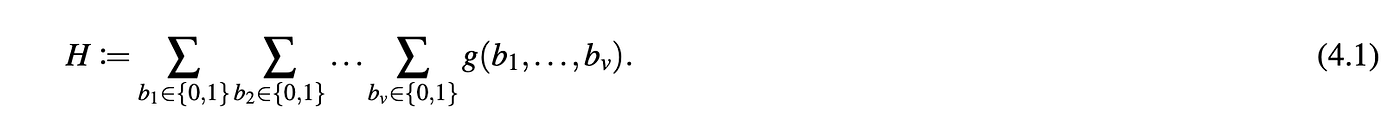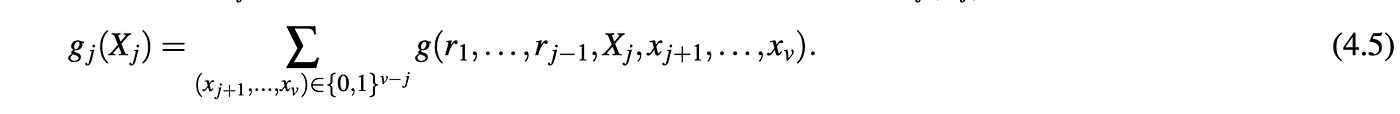# Rust Guide: Sum-Check protocol

## This is an unofficial & unaudited Rust implementation of algorithms in Dr. Thaler’s Proofs, Arguments, & ZK book.

This week we learned about the sum-check protocol. In cases when we want a sum of a polynomial `g` over an exponential size domain, in this case `2^v` (a boolean hypercube), sum-check provides an interactive proof for Provers to claim such sums.

Rather than manually recomputing this sum, the Verifier can just check the Prover’s claim in `O(v + cost-to-compute-g-only-once)` time with only a minor increase in overall Prover runtime.Figure 4.1 in Proofs, Arguments, and Zero-Knowledge

The way I visualize this proof, is that in each round `j`, the Prover sort of affixes one more face of the boolean hypercube with a random `r` given by the Verifier. He then evaluates the sum over the remaining faces of the hypercube, leaving one last edge open for the Verifier to evaluate. This takes the form of a univariate polynomial, which the Verifier can evaluate against the previous round `gj−1(rj−1) = gj(0) + gj(1)`.gj provided by the Prover in each round. Fig 4.5 in Proofs, Arguments, and Zero-Knowledge

Sum-check turns out to be a hammer for a lot of interesting problems.

## Walkthrough

Lets’ walk through this interactive proof and implement it a step at a time using Arkworks ark-poly crate to assist with polynomial representations.

To follow the notation in the following tutorial, please reference starting lemma 4.1 in Dr. Thaler’s book linked here.

The full implementation with tests & benchmarking can be found here:

Necessary caveat: I’m neither a mathematician nor a Rust expert. Suggestions for improvement are always welcome! 🥰

## Round 1

The Prover first sends the Verifier a claimed sum-check value `C1`. Let’s use the following function to represent the Verifier:

`pub fn verify(g: &MultiPoly, c_1: ScalarField) -> bool {TODO}`

Where the type aliases just mean:

• ScalarField: `ark_bls12_381::Fr`
• Multipoly: `SparsePolynomial<ScalarField, SparseTerm>`

Then, the Prover sends the first univariate polynomial g1(X1) claimed to equal C1.g1, a univariate polynomial over X1

To do this, we know that the Prover will have to keep some memory from now on, so let’s define a Prover struct with the following attributes, where:

• `g` is the original polynomial g
• `r_vec` is a growing vector of random elements in a large field provided by the Verifier in each round:

To simulate the Prover calculating `g1`, we implement a function `gen_uni_polynomial.` It computes g over all permutations, all points over the hypercube as follows:

Note: `n_to_vec` transforms a number to its binary representation in a vector format, e.g. 5 => [1,0,1].

Specifically, the function fixes `Xj`, in this case x1, and evaluates over the remaining “unfixed” points of the boolean hypercube. For each point, we compute a univariate polynomial “`unipoly`”. Finally, we sum up all evaluations into a single univariate polynomial.

Stepping one level deeper, it computes each polynomial as follows:

It evaluates `gj` over a vector of points, folding all evaluated terms together into one univariate polynomial at the end.

Stepping one level deeper again, it computes each term as follows, returning a tuple of `(new coefficent, fixed term)`:

In future rounds for example, given a term `x_1²x_2x_3`. If round j= 2, then we leave `x_2` unevaluated (as it will be the variable left to construct the univariate polynomial). `x_1` has been previously fixed by the Verifier, so we replace it with `r_1`. And we evaluate `x_3` over the `point` variable provided by the invoking function.

The verifier invokes the Prover and checks the following:

• C1 = gi(0) +gi(1)
• The degree of `gi` is at most the degree deg_1(g), which is done by constructing a one time look up table `lookup_degree`.

## Intermediate Rounds

For each intermediate round, Verifier chooses a random element r, in our large ScalarField, and sends it to the Prover.

Verifier continues to checks that gj−1(rj−1) = gj(0)+gj(1) holds and that the degrees are still with range.

## Final Round

In the final round, the Verifier chooses a final random element `r` and evaluates the original polynomial g with the `r vector` given to the Prover in throughout the proof.

## Benchmarking

In benchmarking, we isolate the Verifier steps and obtain the following results:

Where:

• `slow_sumcheck_test` tests a Verifier which recomputes the sum
• `sumcheck_test` is our implementation above

Notice the Verifier is linear time. Yay

--

--